# 基于深度学习的推荐系统

2020/11/02 00:03

### 本教程

• 如何使用PyTorch Lightning创建自己的基于深度学习的推荐系统

• 推荐系统中隐式反馈与显式反馈的区别

• 如何在不引入偏差和数据泄漏的情况下训练测试分割数据集以训练推荐系统

• 评估推荐系统的指标（提示：准确度或RMSE不合适！）

### 数据预处理

import pandas as pd
import numpy as np
np.random.seed(123)

rand_userIds = np.random.choice(ratings['userId'].unique(),
size=int(len(ratings['userId'].unique())*0.3),
replace=False)

ratings = ratings.loc[ratings['userId'].isin(rand_userIds)]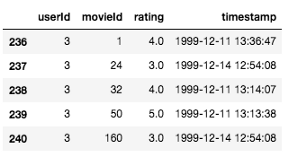#### 训练测试拆分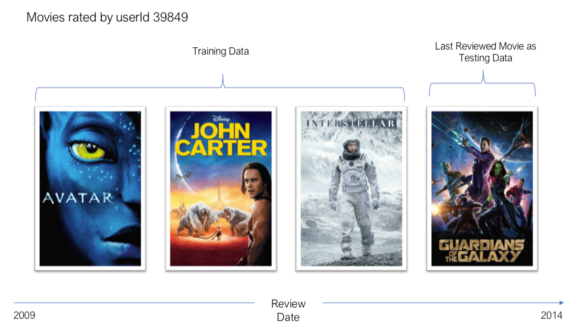ratings['rank_latest'] = ratings.groupby(['userId'])['timestamp'].rank(method='first', ascending=False)

train_ratings = ratings[ratings['rank_latest'] != 1]
test_ratings = ratings[ratings['rank_latest'] == 1]

# 删除我们不再需要的列
train_ratings = train_ratings[['userId', 'movieId', 'rating']]
test_ratings = test_ratings[['userId', 'movieId', 'rating']]


#### 将数据集转换为隐式反馈数据集

train_ratings.loc[:, 'rating'] = 1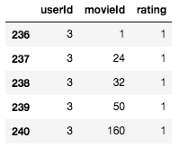# 获取所有电影id的列表
all_movieIds = ratings['movieId'].unique()

# 用于保存训练数据的占位符
users, items, labels = [], [], []

# 这是每个用户都与之交互的项目集
user_item_set = set(zip(train_ratings['userId'], train_ratings['movieId']))

# 4:1
num_negatives = 4

for (u, i) in user_item_set:
users.append(u)
items.append(i)
labels.append(1) # 用户与项目有交互
for _ in range(num_negatives):
# 随机选择一个项目
negative_item = np.random.choice(all_movieIds)
# 检查用户是否与该项目进行了交互
while (u, negative_item) in user_item_set:
negative_item = np.random.choice(all_movieIds)
users.append(u)
items.append(negative_item)
labels.append(0) # 代表没有交互


import torch
from torch.utils.data import Dataset

class MovieLensTrainDataset(Dataset):
"""MovieLens PyTorch数据集用于训练

Args:
ratings (pd.DataFrame): 包含电影评级的DataFrame
all_movieIds (list): 包含所有电影id的列表

"""

def __init__(self, ratings, all_movieIds):
self.users, self.items, self.labels = self.get_dataset(ratings, all_movieIds)

def __len__(self):
return len(self.users)

def __getitem__(self, idx):
return self.users[idx], self.items[idx], self.labels[idx]

def get_dataset(self, ratings, all_movieIds):
users, items, labels = [], [], []
user_item_set = set(zip(ratings['userId'], ratings['movieId']))

num_negatives = 4
for u, i in user_item_set:
users.append(u)
items.append(i)
labels.append(1)
for _ in range(num_negatives):
negative_item = np.random.choice(all_movieIds)
while (u, negative_item) in user_item_set:
negative_item = np.random.choice(all_movieIds)
users.append(u)
items.append(negative_item)
labels.append(0)



### 我们的模型-神经协同过滤（NCF）

#### 用户嵌入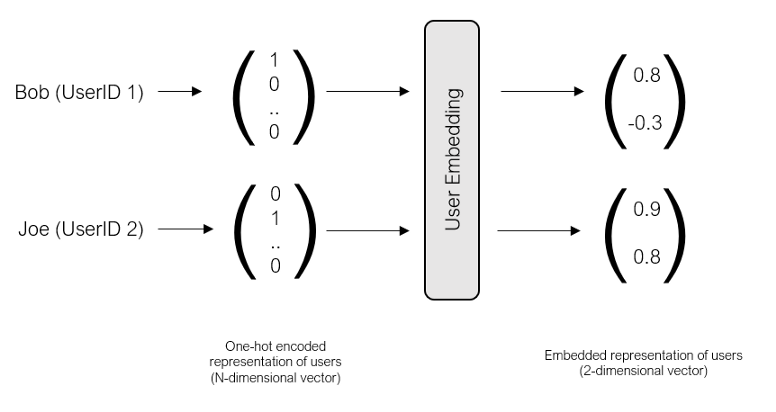#### 模型体系结构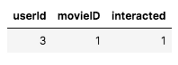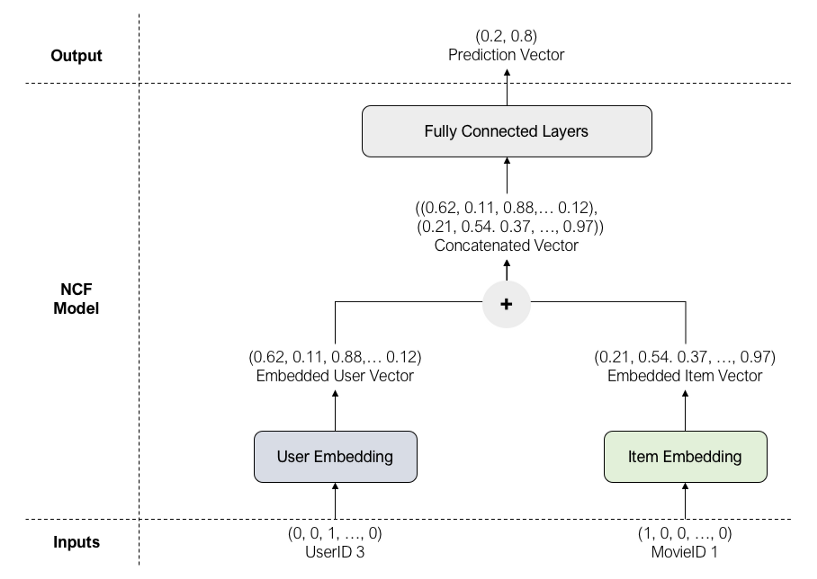import torch.nn as nn
import pytorch_lightning as pl

class NCF(pl.LightningModule):
""" 神经协同过滤(NCF)

Args:
num_users (int): 唯一用户的数量
num_items (int): 唯一项的数量
ratings (pd.DataFrame): 包含用于训练的电影评级
all_movieIds (list): 包含所有movieIds的列表(训练+测试)
"""

def __init__(self, num_users, num_items, ratings, all_movieIds):
super().__init__()
self.user_embedding = nn.Embedding(num_embeddings=num_users, embedding_dim=8)
self.item_embedding = nn.Embedding(num_embeddings=num_items, embedding_dim=8)
self.fc1 = nn.Linear(in_features=16, out_features=64)
self.fc2 = nn.Linear(in_features=64, out_features=32)
self.output = nn.Linear(in_features=32, out_features=1)
self.ratings = ratings
self.all_movieIds = all_movieIds

def forward(self, user_input, item_input):

# 通过嵌入层
user_embedded = self.user_embedding(user_input)
item_embedded = self.item_embedding(item_input)

# Concat两个嵌入层
vector = torch.cat([user_embedded, item_embedded], dim=-1)

# 通过全连接层
vector = nn.ReLU()(self.fc1(vector))
vector = nn.ReLU()(self.fc2(vector))

# 输出层
pred = nn.Sigmoid()(self.output(vector))

return pred

def training_step(self, batch, batch_idx):
user_input, item_input, labels = batch
predicted_labels = self(user_input, item_input)
loss = nn.BCELoss()(predicted_labels, labels.view(-1, 1).float())
return loss

def configure_optimizers(self):

batch_size=512, num_workers=4)


num_users = ratings['userId'].max()+1
num_items = ratings['movieId'].max()+1
all_movieIds = ratings['movieId'].unique()

model = NCF(num_users, num_items, train_ratings, all_movieIds)

progress_bar_refresh_rate=50, logger=False, checkpoint_callback=False)

trainer.fit(model)


### 评估我们的推荐系统• 对于每个用户，随机选择99个用户没有交互的项目。

• 将这99个项目与测试项目（用户最后一次交互的实际项目）结合起来。我们现在有100件。

• 对这100个项目运行模型，并根据它们的预测概率对它们进行排序。

• 从100个项目列表中选择前10个项目。如果测试项出现在前10项中，那么我们认为这是命中。

• 对所有用户重复此过程。命中率就是平均命中率。

#### 命中率@10

# 用于测试的用户-项目对
test_user_item_set = set(zip(test_ratings['userId'], test_ratings['movieId']))

# 每个用户与之交互的所有条目
user_interacted_items = ratings.groupby('userId')['movieId'].apply(list).to_dict()

hits = []
for (u,i) in test_user_item_set:
interacted_items = user_interacted_items[u]
not_interacted_items = set(all_movieIds) - set(interacted_items)
selected_not_interacted = list(np.random.choice(list(not_interacted_items), 99))
test_items = selected_not_interacted + [i]

predicted_labels = np.squeeze(model(torch.tensor([u]*100),
torch.tensor(test_items)).detach().numpy())

top10_items = [test_items[i] for i in np.argsort(predicted_labels)[::-1][0:10].tolist()]

if i in top10_items:
hits.append(1)
else:
hits.append(0)

print("The Hit Ratio @ 10 is {:.2f}".format(np.average(hits)))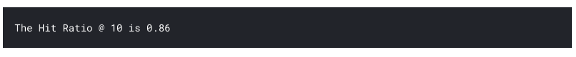### 下一步

sklearn机器学习中文官方文档： http://sklearn123.com/

3
2 收藏

### 作者的其它热门文章0 评论
2 收藏
3